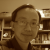# Andy's blog## Farewell 2021 with 3n+1

The Youtube algorithm one day pushed a video "The Simplest Math Problem No One Can Solve - Collatz Conjecture" to me. After viewing the video, I thought that my VRMath2 could easily visualise the 3n+1 graph with its LOGO programing. I am happy that my VRMath2 application is still working after almost three years in low maintenance mode. With some simple coding. I have written a LOGO program that can take a number (natural number) and the turtle will draw its 3n+1 graph. The 3n+1 problem is simple. It starts with a natural number; if the number is odd, multiply it by 3 then add one; if the number is even, the number is divided by 2. These rules apply until the resulting number is one. It is interesting that it seems any given natural number will end with 1 after applying the 3n+1 rules, but how?## Robotic arm

In the last two years or so, I started learning about 3D printing and coding various microcontrollers. The ultimate purpose is to bring these into STEM education and promote maker culture in schools. Last year in 2019, I started a MakerClubQUT and began documenting various maker projects. End of 2019, I started building my first simple robotic arm and thought about the 3D rotations for the robotic arm to reach any particular location in space. I thought that I might try coding a virtual robotic arm in VRMath2, and here it is.## Chaos game - square fractal

Chaos game is a mathematical way to generate fractals. It has a simple algorithm, which (1) starts from a radom point with a polygon, then (2) randomly selects a vertice, (3) plots a new point midway between the starting point and the selected vertice. The ploted mid point then becomes the step (1) and reiterates the steps as many times as you like to generate a geometric figure. The example below in this blog uses a square as the polygon to generate a chaos game square fractal.## The Sun and Earth model

Most of us may know that the distance between the Sun and the Earth is one AU (Astronomical Unit: 150,000,000 km). However, due to the various online solar system images, many of us are not clear about how the Earth and Sun look like in space in the actaul scale. The VRMath2 Editor is a 3D modelling application, and is capable of modelling our solar system. Therefore, I did an experiment in modelling the Earth and Sun using actual parameters. The results are not as good as I expected, but they do make me appreciate the awe of the solar system, and the difficulties in modelling and comprehending the science of our solar system.## Hemnes Desk

The Hemnes desk is a simple design. It has a table top and three compartments for storage. The programming could be simple but because I tool a challenge to use egocentric movements only, so it takes more than two hours to complete. Another reason for the longer time taken was that during the construction, I actually found a bug in the VRMath2 Editor, which puzzled me with some strange behaviour of the turtle movement.